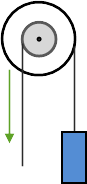# Free ASVAB Practice Tests

• Every Test is Unique
Custom software and unique templates randomize questions, answers, and variables every time you take a new test. You'll never take the same test twice!
• 1,557 Questions, Problems & Flash Cards
Huge database of 668 multiple-choice questions, 135 math and algebra problems, and 754 flash cards to help you prepare for the ASVAB.
• Detailed Solutions
Get a question wrong? All questions and problems have detailed answer explanations so you can learn exactly how to get it right the next time.
• Know You're Ready
Want to know how you stack up? When you're done with a practice test you can compare your score to everyone else who has ever answered those questions.
• Interactive Study Guide
Detailed ASVAB study guide, MOS study guides, and line score study guides outline exactly what you should know to earn your target scores and customized tests and flash cards for each topic let you laser focus your limited study time.
• Brand New for 2019
ASVAB Test Bank has been completely redesigned for 2019 with all new questions, problems, and flash cards. And the redesign isn't done! Coming soon:
More Content
More questions, problems and flash cards
Bookmarks
Create a custom study guide with just the topics you're studying
Score Estimator
Custom estimate of your potential ASVAB score

Plus printable tests, Q&A, and an ad-free upgrade. Have a suggestion? Please let us know what you want!

Take an ASVAB Practice Test

Sample Practice Test Questions

Mechanical ComprehensionIf the radius of the axle is 5 and the radius of the wheel is 8, what is the mechanical advantage of this wheel and axle configuration?
0.63

The mechanical advantage of a wheel and axle is the input radius divided by the output radius:

MA = $$\frac{r_i}{r_o}$$

In this case, the input radius (where the effort force is being applied) is 5 and the output radius (where the resistance is being applied) is 8 for a mechanical advantage of $$\frac{5}{8}$$ = 0.63

Word Knowledge
Dubious most nearly means:
suspect
Questionable.
Mechanical Comprehension

For a hydraulic system, pressure applied to the input of the system will increase the pressure in which parts of the system?

all of these are correct

Pascal's law states that a pressure change occurring anywhere in a confined incompressible fluid is transmitted throughout the fluid such that the same change occurs everywhere. For a hydraulic system, this means that a pressure applied to the input of the system will increase the pressure everywhere in the system.

Word Knowledge
Rehash most nearly means:
review
To discuss again.
Automotive Information

Ignition timing defines the point in time at the end of the compression stroke that which of the following happens?

spark plugs fire

Ignition timing defines the point in time at the end of the compression stroke that the spark plug fires. Measured in number of degrees before top dead center (BTDC), the exact point that the spark plugs initiate combustion varies depending on the speed of the engine. The timing is advanced (the spark plugs fire a few more degrees BTDC) when the engine is running faster and retarded when it's running slower.

General Science

Which of the following is not part of the carbon cycle?

precipitation

The carbon cycle represents the ciruit of carbon through Earth's ecosystem. Carbon dioxide (CO2) in the atmosphere is absorbed by plants through photosynthesis. Plants then die and release carbon back into the atmosphere during decomposition or are eaten by animals who breathe (respiration) the carbon into the atmosphere they exhale and produce waste which also releases carbon as it decays.

Mechanical Comprehension

A block and tackle with four pulleys would have a mechanical advantage of:

4

Two or more pulleys used together constitute a block and tackle which, unlike a fixed pulley, does impart mechanical advantage as a function of the number of pulleys that make up the arrangement.  So, for example, a block and tackle with three pulleys would have a mechanical advantage of three.

Word Knowledge
The best–selling writer was disappointed; his new novel was only a moderate success.
medium
Tending toward the average.
Word Knowledge
Periodic most nearly means:
repeated
Occurring at regular intervals.
Arithmetic Reasoning

What is $$\frac{4}{6}$$ + $$\frac{4}{14}$$?

$$\frac{20}{21}$$

To add these fractions, first find the lowest common multiple of their denominators. The first few multiples of 6 are [6, 12, 18, 24, 30, 36, 42, 48, 54, 60] and the first few multiples of 14 are [14, 28, 42, 56, 70, 84, 98]. The first few multiples they share are [42, 84] making 42 the smallest multiple 6 and 14 share.

Next, convert the fractions so each denominator equals the lowest common multiple:

$$\frac{4 x 7}{6 x 7}$$ + $$\frac{4 x 3}{14 x 3}$$

$$\frac{28}{42}$$ + $$\frac{12}{42}$$

Now, because the fractions share a common denominator, you can add them:

$$\frac{28 + 12}{42}$$ = $$\frac{40}{42}$$ = $$\frac{20}{21}$$